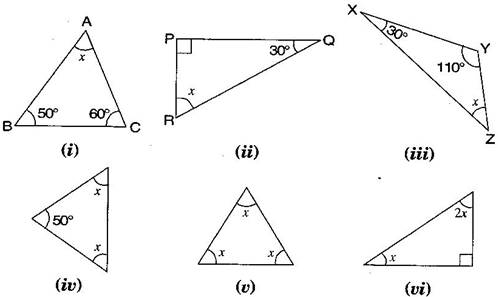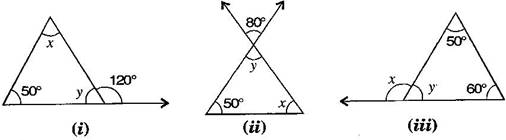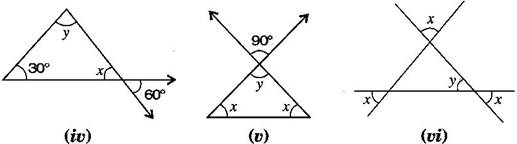### The Triangle and its Properties - Solutions 3

CBSE Class –VII Mathematics
NCERT Solutions
Chapter 6 The Triangle and its Properties
(Ex. 6.3)

Question 1. Find the value of unknown $x$ in the following diagrams:Answer: (i) In $\mathrm{\Delta }\text{ABC},$
$\mathrm{\angle }$BAC + $\mathrm{\angle }$ABC + $\mathrm{\angle }$ACB = ${180}^{\circ }$ [By angle sum property of a triangle]
$⇒$ $x+{50}^{\circ }+{60}^{\circ }={180}^{\circ }$
$⇒$ $x+{110}^{\circ }={180}^{\circ }$ $⇒$ $x={180}^{\circ }-{110}^{\circ }={70}^{\circ }$
(ii) In $\mathrm{\Delta }$PQR,
$\mathrm{\angle }$RPQ + $\mathrm{\angle }$PQR + $\mathrm{\angle }$RPQ = ${180}^{\circ }$ [By angle sum property of a triangle]
$⇒$ ${90}^{\circ }+{30}^{\circ }+x={180}^{\circ }$
$⇒$ $x+{120}^{\circ }={180}^{\circ }$ $⇒$ $x={180}^{\circ }-{120}^{\circ }={60}^{\circ }$
(iii) In $\mathrm{\Delta }$XYZ,
$\mathrm{\angle }$ZXY + $\mathrm{\angle }$XYZ + $\mathrm{\angle }$YZX = ${180}^{\circ }$ [By angle sum property of a triangle]
$⇒$ ${30}^{\circ }+{110}^{\circ }+x={180}^{\circ }$
$⇒$ $x+{140}^{\circ }={180}^{\circ }$ $⇒$ $x={180}^{\circ }-{140}^{\circ }={40}^{\circ }$
(iv) In the given isosceles triangle,
$x+x+{50}^{\circ }={180}^{\circ }$ [By angle sum property of a triangle]
$⇒$ $2x+{50}^{\circ }={180}^{\circ }$
$⇒$ $2x={180}^{\circ }-{50}^{\circ }$ $⇒$ $2x={130}^{\circ }$
$⇒$ $x=\frac{{130}^{\circ }}{2}={65}^{\circ }$
(v) In the given equilateral triangle,
$x+x+x={180}^{\circ }$ [By angle sum property of a triangle]
$⇒$ $3x={180}^{\circ }$
$⇒$ $x=\frac{{180}^{\circ }}{3}={60}^{\circ }$
(vi) In the given right angled triangle,
$x+2x+{90}^{\circ }={180}^{\circ }$ [By angle sum property of a triangle]
$⇒$ $3x+{90}^{\circ }={180}^{\circ }$
$⇒$ $3x={180}^{\circ }-{90}^{\circ }$ $⇒$ $3x={90}^{\circ }$
$⇒$ $x=\frac{{90}^{\circ }}{3}={30}^{\circ }$
Question 2. Find the values of the unknowns $x$ and $y$ in the following diagrams:Answer: (i) ${50}^{\circ }+x={120}^{\circ }$ [Exterior angle property of a $\mathrm{\Delta }$ ]
$⇒$ $x={120}^{\circ }-{50}^{\circ }={70}^{\circ }$
Now, ${50}^{\circ }+x+y={180}^{\circ }$ [Angle sum property of a $\mathrm{\Delta }$ ]
$⇒$ ${50}^{\circ }+{70}^{\circ }+y={180}^{\circ }$
$⇒$ ${120}^{\circ }+y={180}^{\circ }$ $⇒$ $y={180}^{\circ }-{120}^{\circ }={60}^{\circ }$
(ii) $y={80}^{\circ }$ ……….(i) [Vertically opposite angle]
Now, ${50}^{\circ }+x+y={180}^{\circ }$ [Angle sum property of a $\mathrm{\Delta }$ ]
$⇒$ ${50}^{\circ }+{80}^{\circ }+x={180}^{\circ }$
[From eq. (i)]
$⇒$ ${130}^{\circ }+x={180}^{\circ }$ $⇒$ $x={180}^{\circ }-{130}^{\circ }={50}^{\circ }$
(iii) ${50}^{\circ }+{60}^{\circ }=x$ [Exterior angle property of a $\mathrm{\Delta }$ ]
$⇒$ $x={110}^{\circ }$
Now ${50}^{\circ }+{60}^{\circ }+y={180}^{\circ }$ [Angle sum property of a $\mathrm{\Delta }$ ]
$⇒$ ${110}^{\circ }+y={180}^{\circ }$
$⇒$ $y={180}^{\circ }-{110}^{\circ }$ $⇒$ $y={70}^{\circ }$
(iv) $x={60}^{\circ }$ ……….(i) [Vertically opposite angle]
Now, ${30}^{\circ }+x+y={180}^{\circ }$ [Angle sum property of a $\mathrm{\Delta }$ ]
$⇒$ ${30}^{\circ }+{60}^{\circ }+y={180}^{\circ }$ [From eq. (i)]
$⇒$ ${90}^{\circ }+y={180}^{\circ }$ $⇒$ $y={180}^{\circ }-{90}^{\circ }={90}^{\circ }$
(v) $y={90}^{\circ }$ ……….(i) [Vertically opposite angle]
Now, $y+x+x={180}^{\circ }$ [Angle sum property of a $\mathrm{\Delta }$ ]
$⇒$ ${90}^{\circ }+2x={180}^{\circ }$ [From eq. (i)]
$⇒$ $2x={180}^{\circ }-{90}^{\circ }$ $⇒$ $2x={90}^{\circ }$
$⇒$ $x=\frac{{90}^{\circ }}{2}={45}^{\circ }$
(vi) $x=y$ ……….(i) [Vertically opposite angle]
Now, $x+x+y={180}^{\circ }$ [Angle sum property of a $\mathrm{\Delta }$ ]
$⇒$ $2x+x={180}^{\circ }$ [From eq. (i)]
$⇒$ $3x={180}^{\circ }$ $⇒$